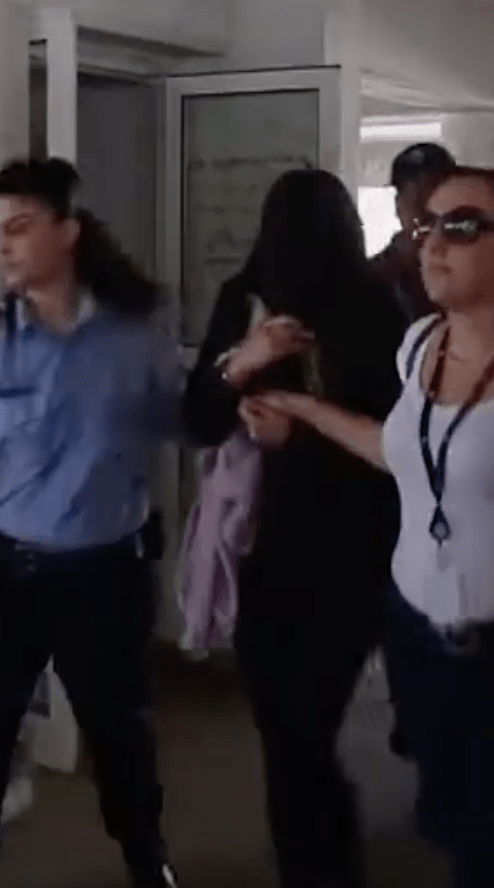# Was woman who made false rape claim against Israelis in Cyprus, ANTIFA?

Video of woman arriving in court:

=<=<=<=<=<=<=<=<=<=<=<=<=<=<=

Black hoodie and leggings? Seems kinda ANTIFA uniform standard to me.

Lots of cameras in front of her. Hopefully some of those will turn up soon.

And it did!

=<=<=<=<=<=<=<=<=<=<=<=<=<=<=Thank you M., ML. for this one.1.Richard on said: